# KSEEB Solutions for Class 6 Maths Chapter 13 Symmetry Ex 13.1

Students can Download Chapter 13 Symmetry Ex 13.1 Questions and Answers, Notes Pdf, KSEEB Solutions for Class 6 Maths helps you to revise the complete Karnataka State Board Syllabus and score more marks in your examinations.

## Karnataka State Syllabus Class 6 Maths Chapter 13 Symmetry Ex 13.1

Question 1.
List any four Symmetrical objects from your home or School?
Solution:
Paper, Sheet, Glass, Cd, Bucket.

Question 2.
For the given figure, Which one is the mirror line, l1 or l2 ?
Solution:Line l2 is the mirror line of this figure. This is because When the given figure is folded about the line l2, the left part can exactly cover the right part and vice – versa.Question 3.
Identify the shapes given below. Check whether they are symmetric or not. Draw the line of symmetry as well.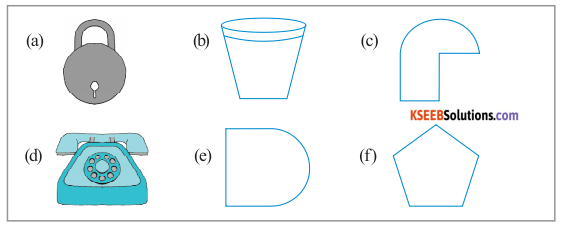Solution:
a) Yes
b) Yes
c) No
d) Yes
e) Yes
f) YesQuestion 4.
Copy the following on a squared paper. A square paper is What you would have used in your arithmetic note book in earlier classes. Then complete them such that the dotted line is the line of symmetry?Solution: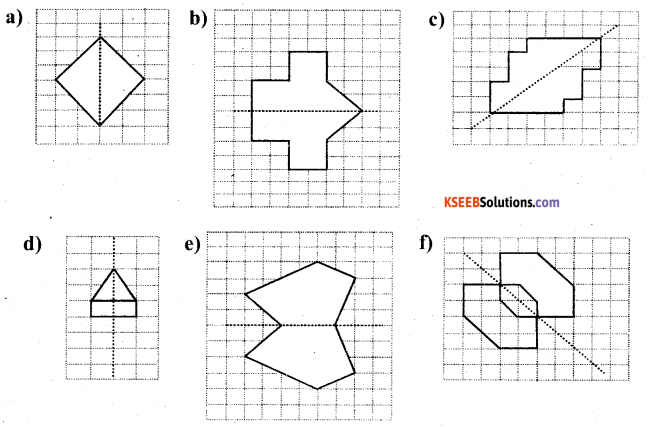Question 5.
In the figure, l is the line of symmetry. Complete the diagram to make it symmetric?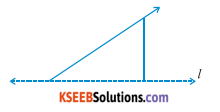Solution:
To make the diagram symmetric, it can be completed as follows.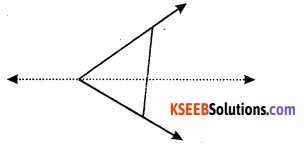Question 6.
In the figure, l is the line of symmetry. Draw the image of the triangle and complete
the diagram so that it becomes symmetric?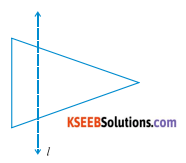Solution:
The required triangle can be formed as followserror: Content is protected !!